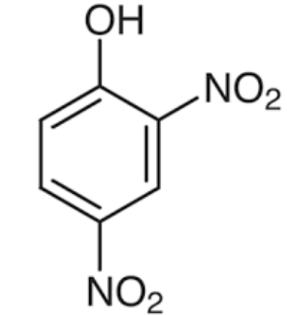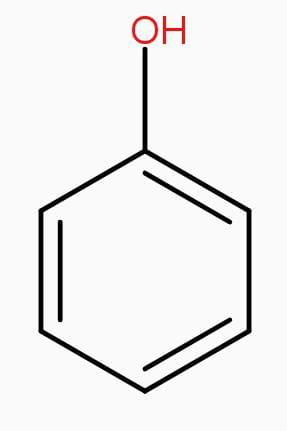QuestionAnswers

# Unlike phenol, 2,4-dinitrophenol is soluble in sodium carbonate solution in water because?A. presence of two - $N{{O}_{2}}$groups in the ring makes 2, 4 – dinitrophenol a stronger acid than phenol.B. presence of two - $N{{O}_{2}}$groups in the ring makes 2, 4 – dinitrophenol a weaker acid than phenol.C. presence of two - $N{{O}_{2}}$groups make the hydrogen bonding easier, making 2,4-dinitrophenol soluble.D. nitro group reacts with $N{{a}_{2}}C{{O}_{3}}$while the -OH group does not.

Hint: To solve this question you should have a basic idea regarding the structures of phenol and 2,4-dinitrophenol and about electron withdrawing groups and its effect after adding to any compound. Phenol has a benzene ring with one hydroxyl group and 2,4-dinitrophenol has two nitrogen oxide molecules more than phenol.

Structures of phenol and 2,4-dinitrophenol are drawn below.(2,4 – dinitrophenol) (phenol)
In 2,4 – dinitrophenol two - $N{{O}_{2}}$ groups are present. $N{{O}_{2}}$ has a strong electron withdrawing nature which increases acidity of the compound.
Electron withdrawing group (EWG): An atom or group that draws electron density from neighboring atoms towards itself, usually by resonance or inductive effects.
Therefore, presence of two electron withdrawing - $N{{O}_{2}}$groups in the ring makes 2,4- dinitrophenol a stronger acid than phenol.
Sodium Carbonate solution is basic in nature. Hence, Stronger the acid it is easily soluble in the basic solution.
Therefore, it reacts with $N{{a}_{2}}C{{O}_{3}}$solution to form sodium salt with the evolution of $C{{O}_{2}}$, thus making it soluble in$N{{a}_{2}}C{{O}_{3}}$.
Hence, unlike phenol, 2,4-dinitrophenol is soluble in sodium carbonate solution in water because presence of two - $N{{O}_{2}}$groups in the ring makes 2, 4 – dinitrophenol a stronger acid than phenol.

Hence, option A is the right answer.
Note:
Always remember, 2,4,6 – trinitrophenol > 2,4 – dinitrophenol > phenol, order of acidic nature of the compounds.
Orders of electron withdrawing group is -$N{{O}_{2}}$, -$CN$,-$CHO$,-$COR$,-$COOH$,-$COOR$, -$CON{{H}_{2}}$(strongest to weakest)